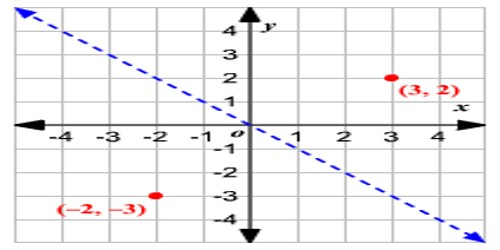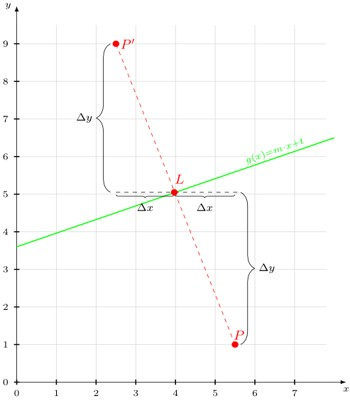Reflection of a Point in a Line

A reflection is a transformation representing a flip of a figure. Figures may be reflected in a point, a line, or a plane. When reflecting a figure in a line or in a point, the image is congruent to the preimage.

A reflection maps every point of a figure to an image across a fixed line. The fixed line is called the line of reflection.

A reflection of a point over the line y = − x

y = −x is shown.The rule for a reflection in the origin is (x, y) → (− y, − x)

Explanation

It’s astonishing how difficult it is to find a good explanation how to reflect a point over a line that does not use higher math methods. So here is my explanation:

You have a point, P = (x, y) and a line g(x) = m⋅x + t and you want to get the point

P ′= (x′, y′) that got mirrored over gAs you can see, you can construct this quite easily on paper:

• Construct the perpendicular through P to g. It starts in L and ends in P.
• Double the length of the perpendicular in the direction of L.
• The endpoint is P′.

How can you do that without drawing it?

First you have to get the perpendicular

s (x) = ms⋅x + t (the dashed red line).

You have to know this:

ms = −1/m And then you know that P is on s. So you simply put in the values (x, y) of P and solve to t : t = y − ms ⋅ x

Now you have s. As s and g have exactly point in common, the following equation gives exactly one result:

s (x) = g (x)

You have to solve for x. Then you only need to put x into s (x) or g (x) and you’re done. You’ve calculated L = (x, y).

Now you know Δx = |xL – xP| and

Δy = |yL –yP| and you can calculate P′.

Information Source: